# Lions Weekly: February 2, 2022# A Note From Mr. Sandefur

Lion Family,

As we are fast approaching the end of our priority re-enrollment window, I want to thank everyone who has already informed us of your intent for next year and would like to encourage those who have not yet to please remember to do so by the end of the day on Friday. Our leadership team can begin planning in earnest for the next academic year with this information, and for that, we are truly grateful. As I spent some time reflecting on the past several months, the one thing that has stood out to me the most is that TKA is an exceptional place. Our families, faculty, and we share a common desire to see Christ honored in all we do. As we seek to approach this spring semester, please join with me in praying that the presence of the Lord is here in this place.

In Christ Alone,

Jeremy Sandefur
President

 table div table+table+table+table div table{width:100%;padding:0}table div table+table+table+table div table img{width:96.23%;padding:0;float:none}table div table+table+table+table div table td{width:100%;padding:0 1.88% 18px}/* styles */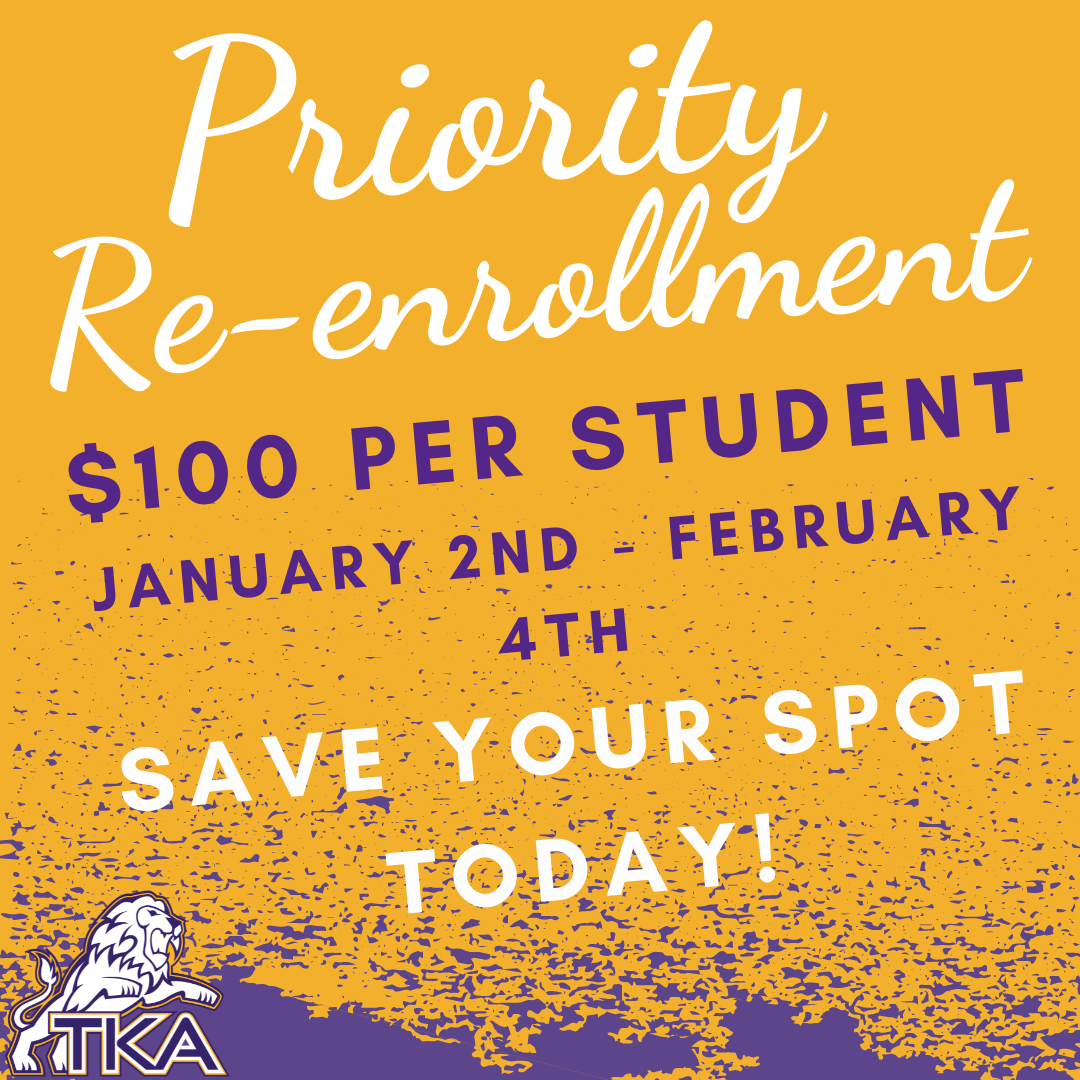# Re-enrollment for 2022-2023

Re-enrollment packets are live in the FACTS Family Portal!

Priority re-enrollment fee of \$100 per student (non-refundable, guarantees your child’s spot) will end on Friday, February 4th.

Re-enrollment packets submitted after February 4th will carry a fee of \$300 per student.

The following are to be submitted:
• Re-enrollment form (through FACTS)
• Payment (any of the following options are acceptable):
1. Cash (submitted to either grade level office)
2. Check (submitted to either grade level office)
3. Payment through FACTS account (families will receive more information regarding how to pay this way. This allows for the use of a card or bank draft as a payment option).

If you have a sibling that will be new to TKA for the 2022-2023 school year, please contact Lori Campbell to begin the application process.

Lori Campbell
(865) 573-8321 option 1

 table div table+table+table+table+table+table div table{width:100%;padding:0}table div table+table+table+table+table+table div table img{width:96.23%;padding:0;float:none}table div table+table+table+table+table+table div table td{width:100%;padding:0 1.88% 18px}/* styles */# Pajama Dress Up Day

## Thursday, February 3

Jr. Beta will be hosting the pajama dress up day to continue partnering with and raising funds for the Isaiah 117 House as we continue to Love Where We Live.
For the donation of \$1.00 (or more) per dress up day, students can wear this fun attire. Please remember that all attire must adhere to dress code.

 table div table+table+table+table+table+table+table+table div table{width:100%;padding:0}table div table+table+table+table+table+table+table+table div table img{width:96.23%;padding:0;float:none}table div table+table+table+table+table+table+table+table div table td{width:100%;padding:0 1.88% 18px}/* styles */# Friday, February 4, Dress Up Day## Elementary School

Elementary students will dress in their Jr. League attire to celebrate this great season! Remember, all attire must adhere to dress code.

Elementary Parents: You're invited to attend our elementary Jr. League pep rally on Friday, February 4, at 2:00 p.m. in the Tibbetts Gym. We will be celebrating our Little Lions' great Jr. League season!## Middle and High School

In honor of basketball's senior night, middle and high school students can dress in your best "senior" attire. Remember, all attire must adhere to dress code.

 table div table+table+table+table+table+table+table+table+table+table+table+table div table{width:100%;padding:0}table div table+table+table+table+table+table+table+table+table+table+table+table div table img{width:96.23%;padding:0;float:none}table div table+table+table+table+table+table+table+table+table+table+table+table div table td{width:100%;padding:0 1.88% 18px}/* styles */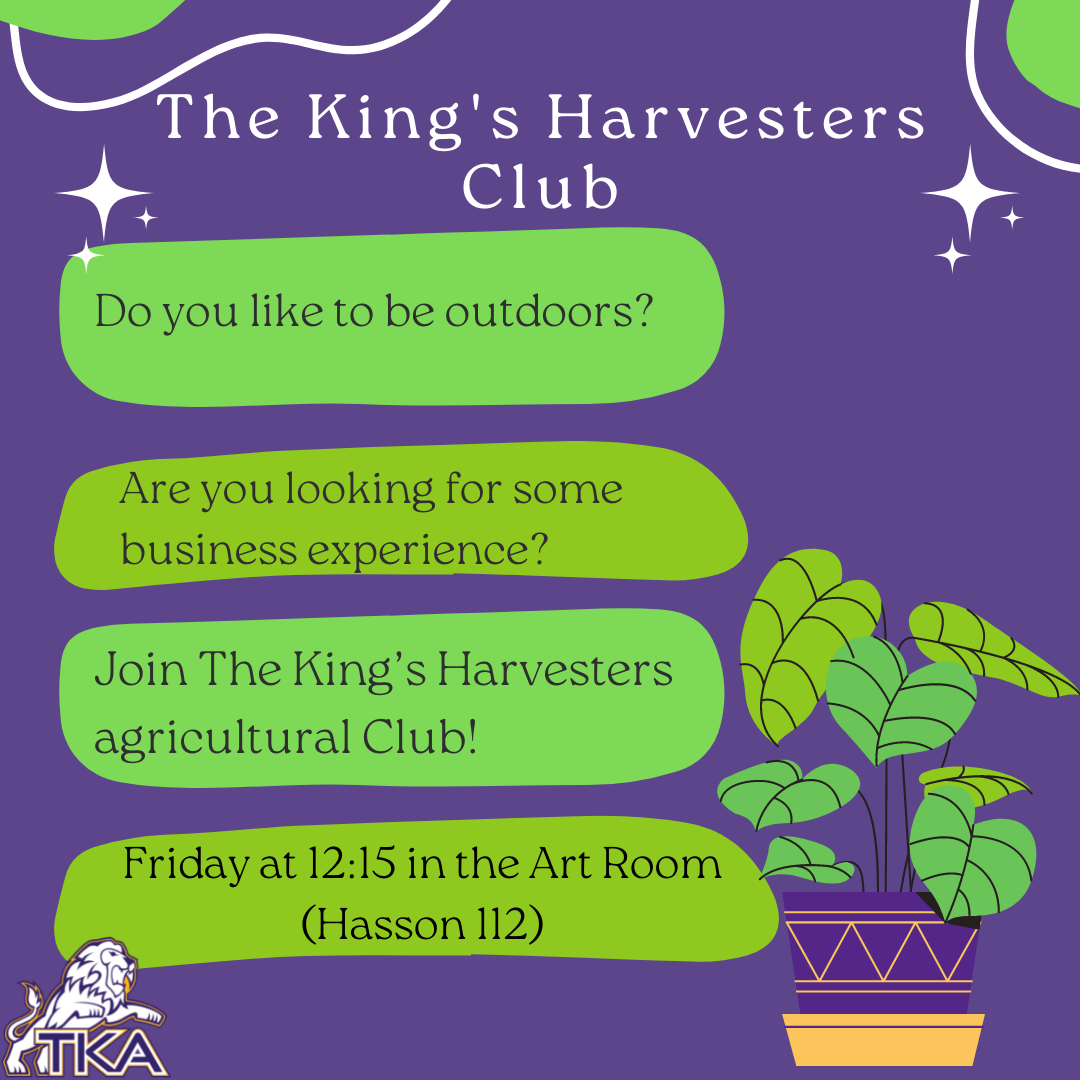# Middle and High School Club

TKA is proud to introduce a new club for Middle and High School students - The King’s Harvesters! Do you like to be outdoors? Are you looking for some business experience? Join The King’s Harvesters!

Students will use the school greenhouse and garden to learn how to grow their own food! Gain experience with the entire agricultural cycle, from planting, to harvesting, to selling goods at a farmer’s market.

Students who are interested should come to a short meeting with Mrs. Rodriguez and Mrs. Smith this Friday at 12:15 in the Art Room (Hasson 112). This will be during the Middle School Power Hour, and at the beginning of High School lunch.

Parents are welcome to get involved as well! Parents who are interested may email Mrs. Rodriguez at krodriguez@thekingsacademy.net for more information.

 table div table+table+table+table+table+table+table+table+table+table+table+table+table+table div table{width:100%;padding:0}table div table+table+table+table+table+table+table+table+table+table+table+table+table+table div table img{width:96.23%;padding:0;float:none}table div table+table+table+table+table+table+table+table+table+table+table+table+table+table div table td{width:100%;padding:0 1.88% 18px}/* styles */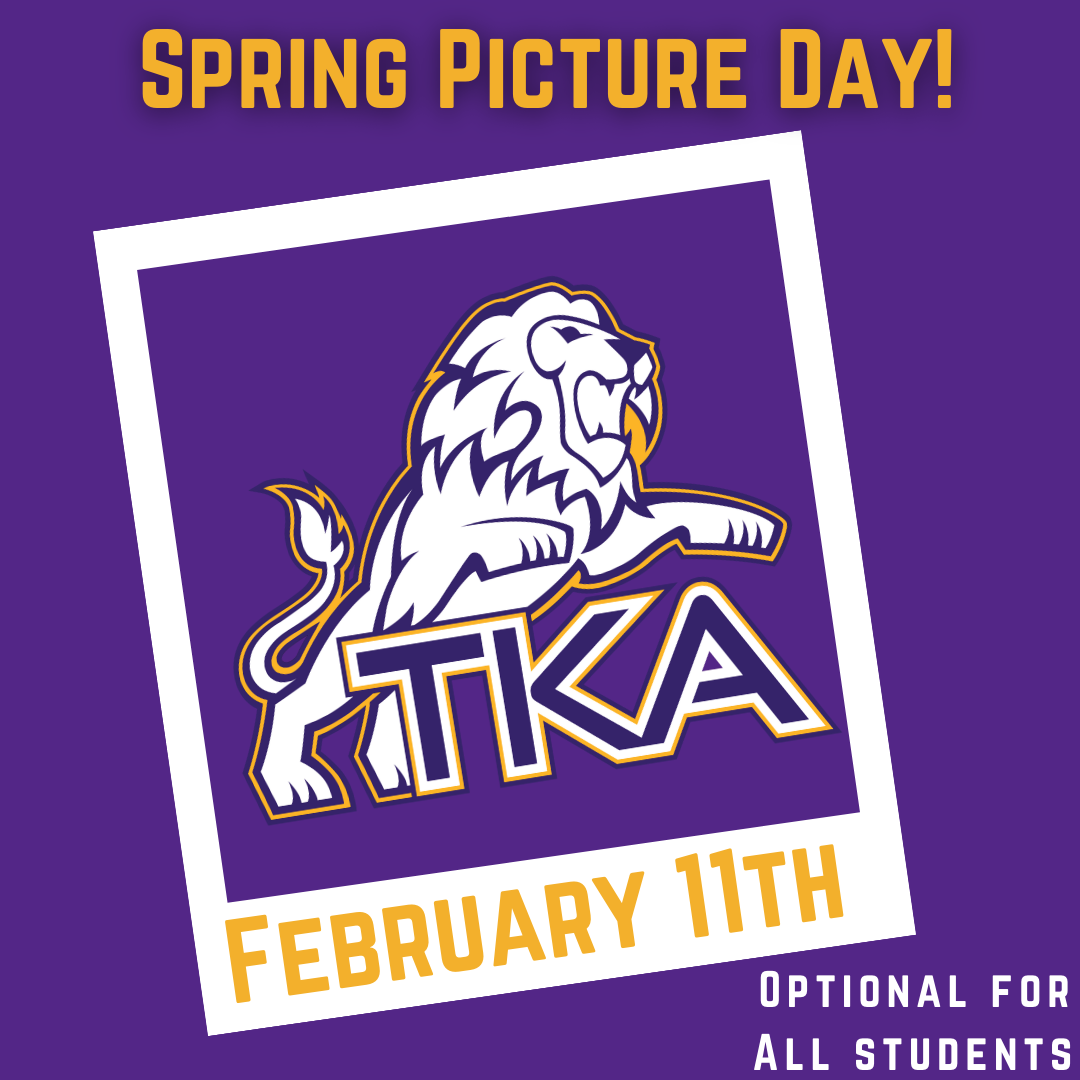# Spring Picture Day

## Friday, February 11

Spring picture day is optional for any student who chooses to participate. Picture package options will be sent home with more information.

 table div table+table+table+table+table+table+table+table+table+table+table+table+table+table+table+table div table{width:100%;padding:0}table div table+table+table+table+table+table+table+table+table+table+table+table+table+table+table+table div table img{width:96.23%;padding:0;float:none}table div table+table+table+table+table+table+table+table+table+table+table+table+table+table+table+table div table td{width:100%;padding:0 1.88% 18px}/* styles */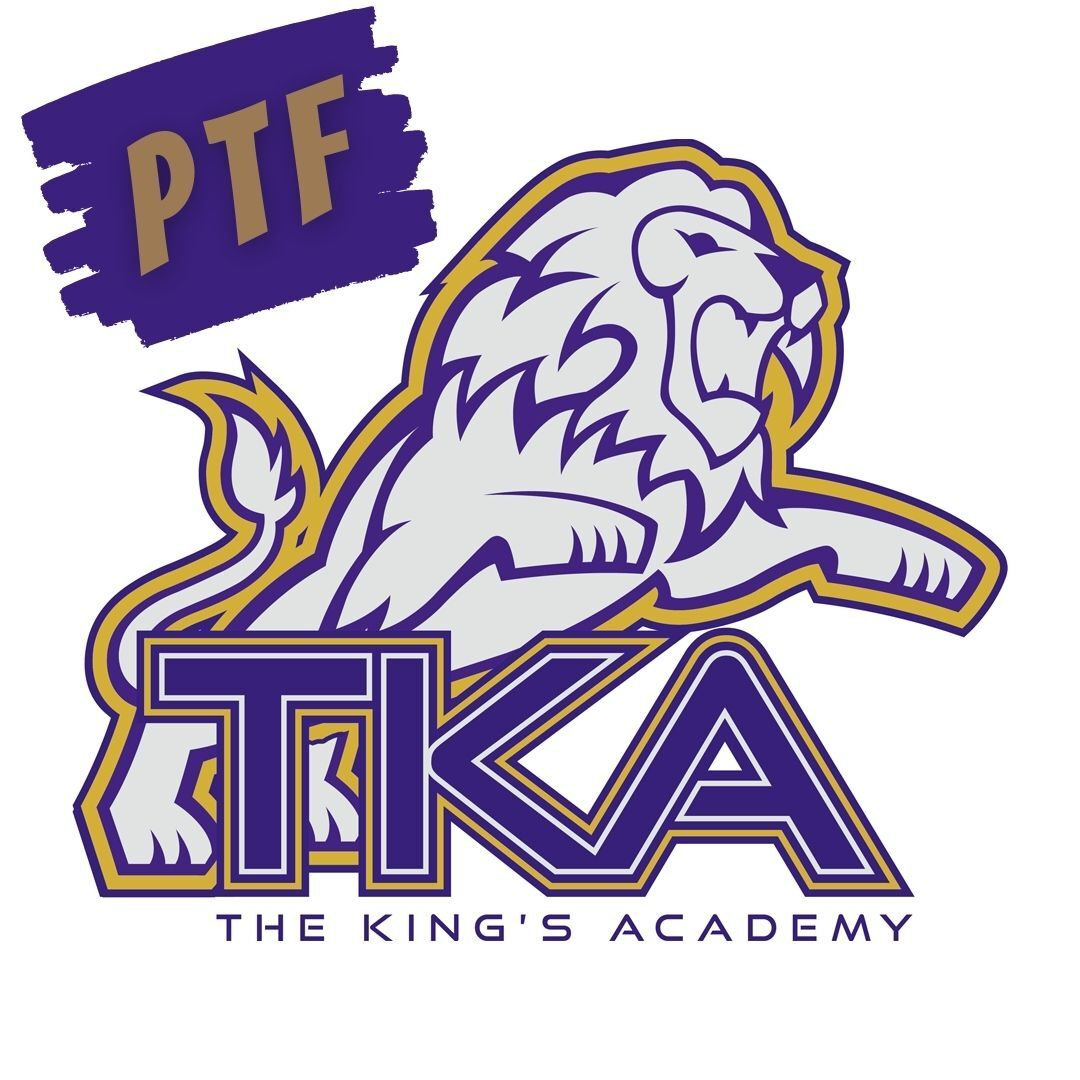# PTF

The next PTF meeting will be Thursday, February 17, from 3:15 PM-4:05 PM in Brandes.

Childcare will be provided throughout the aftercare program free of charge until 4:15 PM for the meeting.

If you haven't joined PTF, no worries, come anyway! Membership forms will be available at the meeting. The fee to join is \$25 per family.

We look forward to having you as part of our PTF this year!

 table div table+table+table+table+table+table+table+table+table+table+table+table+table+table+table+table+table+table div table{width:100%;padding:0}table div table+table+table+table+table+table+table+table+table+table+table+table+table+table+table+table+table+table div table img{width:96.23%;padding:0;float:none}table div table+table+table+table+table+table+table+table+table+table+table+table+table+table+table+table+table+table div table td{width:100%;padding:0 1.88% 18px}/* styles */# Save The Date

TKA will be hosting the “Forged Parent Conference” on March 5th in Woody Auditorium. “Forged” will be a morning of biblically-based parenting seminars focused on encouraging parents that are raising students in a post-Christian society. We are blessed to have local ministers from our community coming to share on topics involving discipleship, maneuvering technology in today’s digital society, and student identity in an ever-changing environment. This event is free and will be a great opportunity to link arms with other parents as we focus on what the Bible says about the roles and responsibilities associated with raising children. Join us from 9:00 – 12:00 on Saturday, March 5th for “Forged!”

 table div table+table+table+table+table+table+table+table+table+table+table+table+table+table+table+table+table+table+table+table div table{width:100%;padding:0}table div table+table+table+table+table+table+table+table+table+table+table+table+table+table+table+table+table+table+table+table div table img{width:96.23%;padding:0;float:none}table div table+table+table+table+table+table+table+table+table+table+table+table+table+table+table+table+table+table+table+table div table td{width:100%;padding:0 1.88% 18px}/* styles */# Upcoming Weekly Sporting Events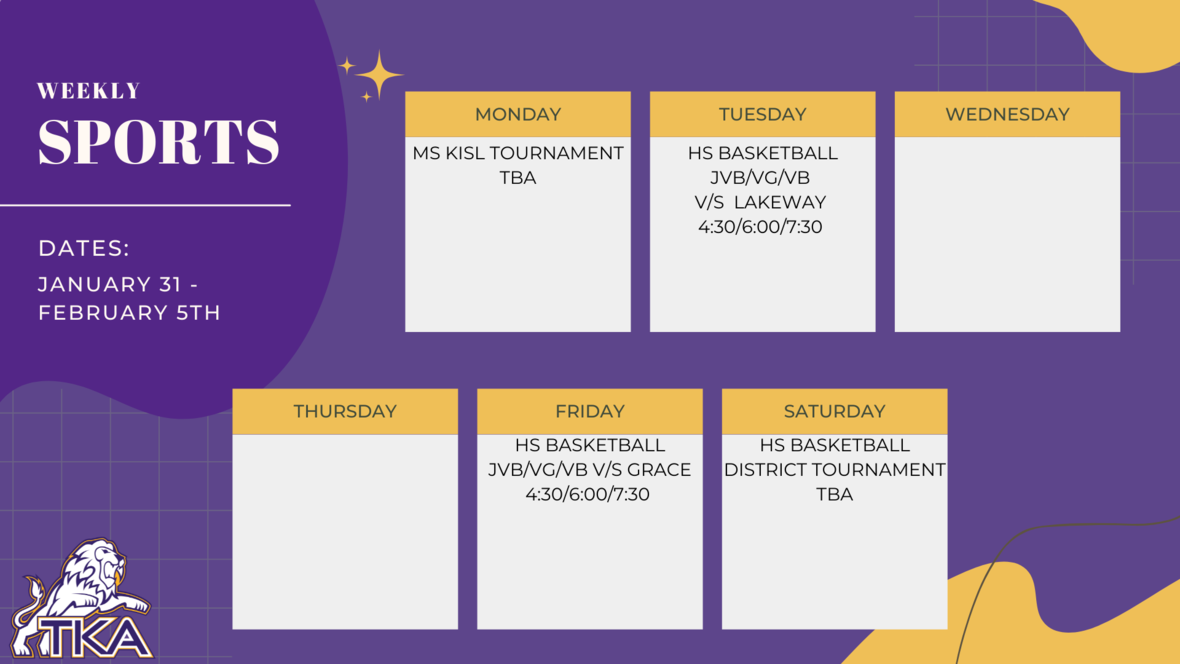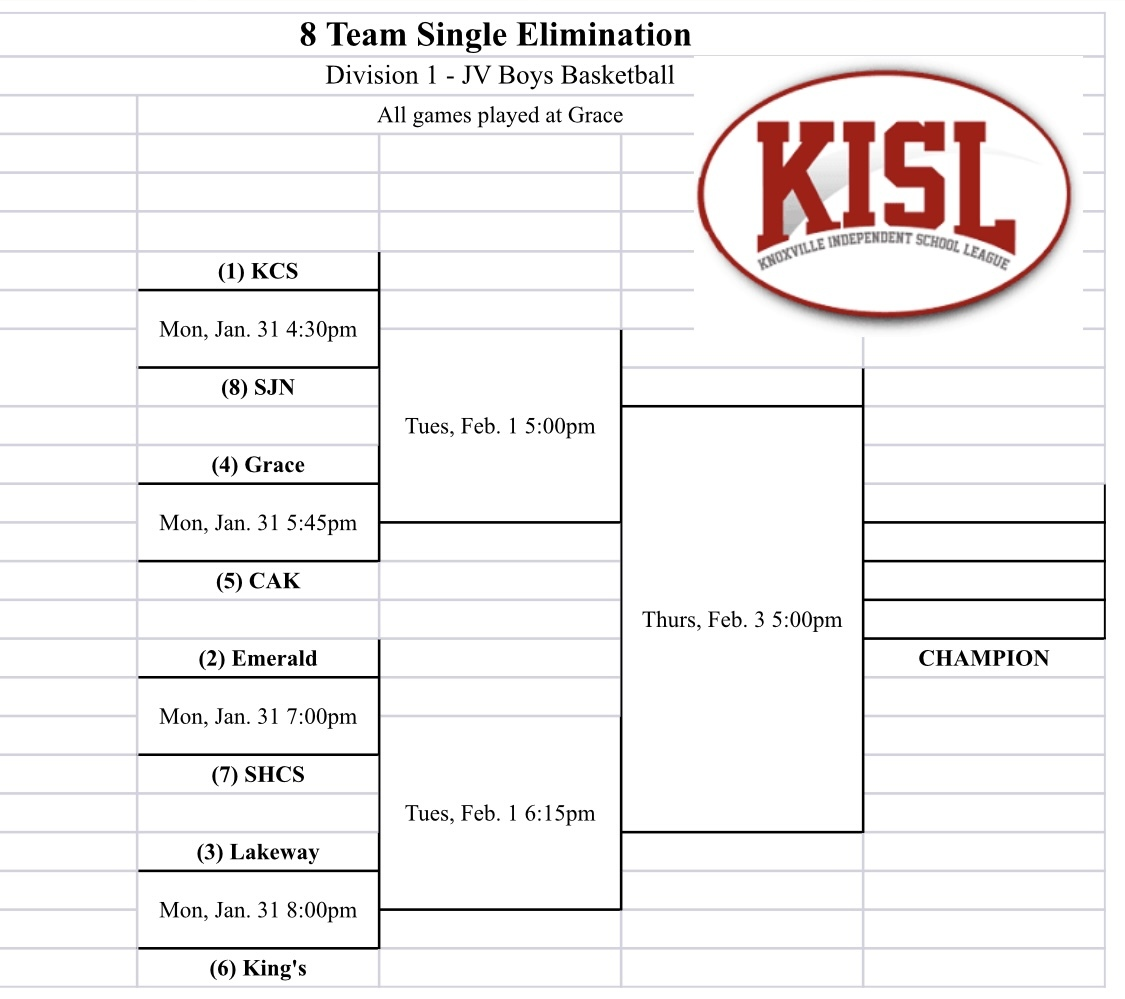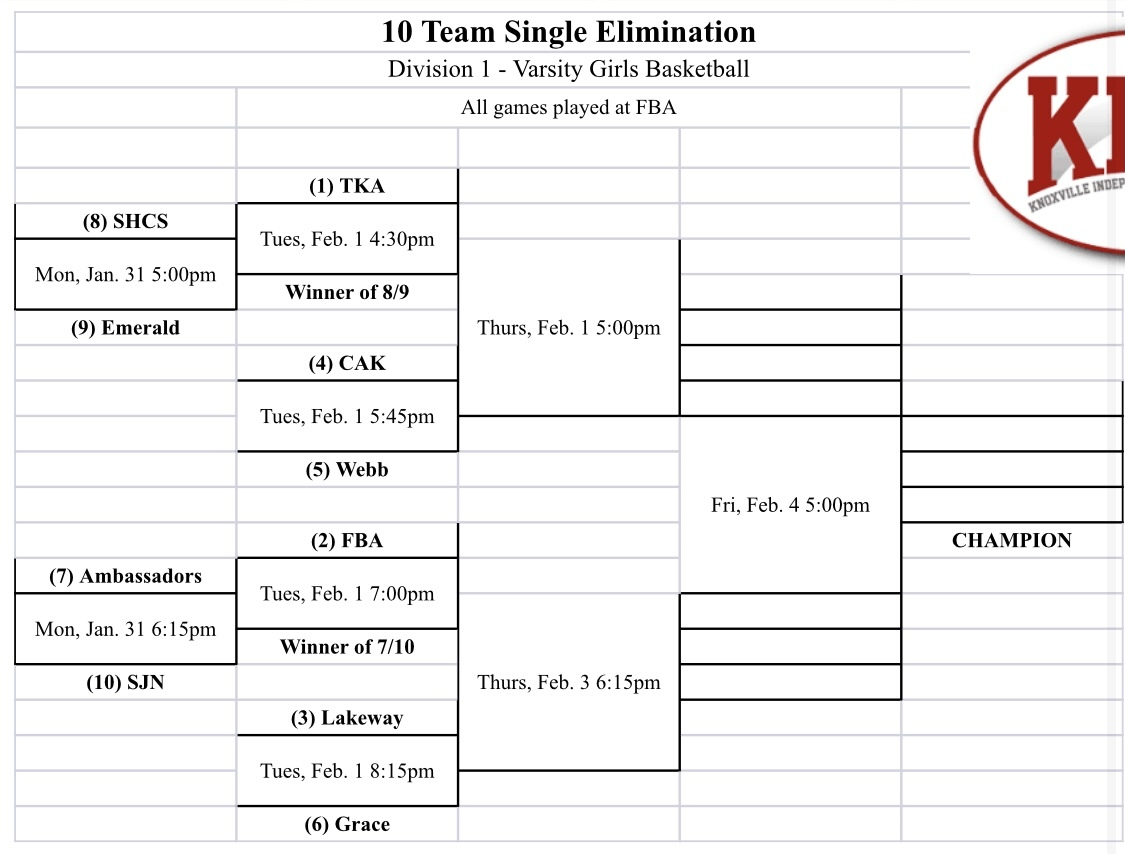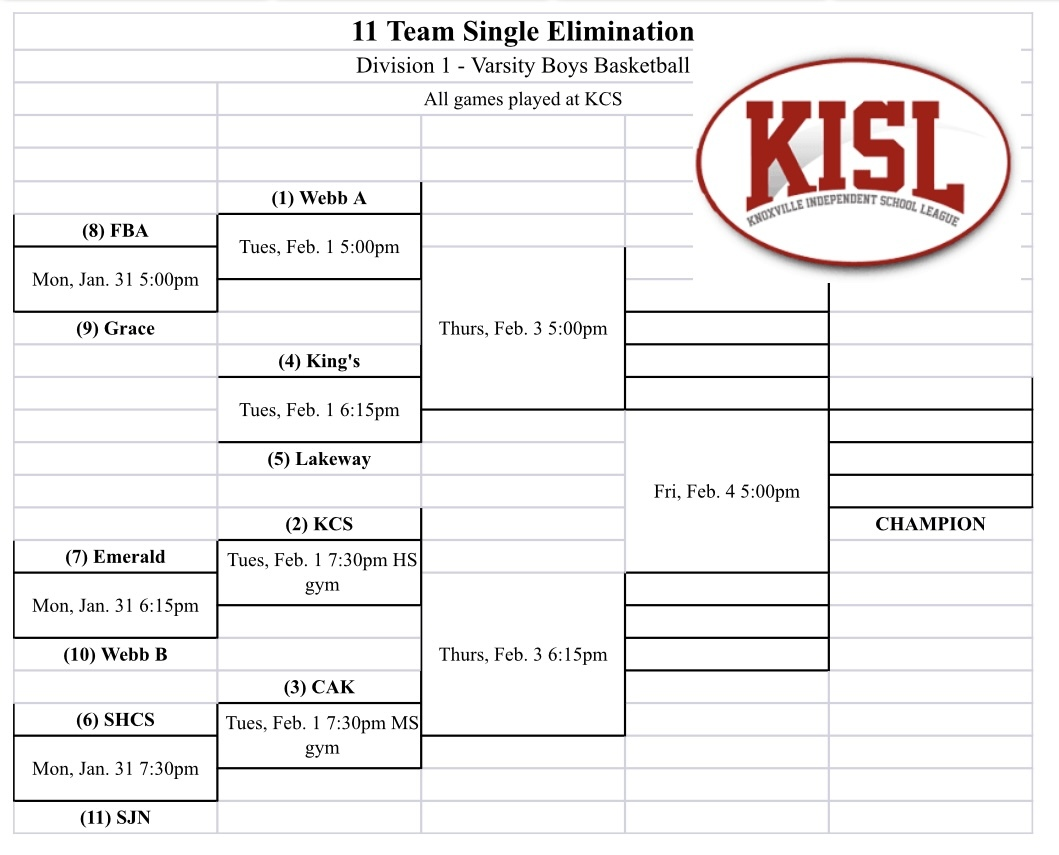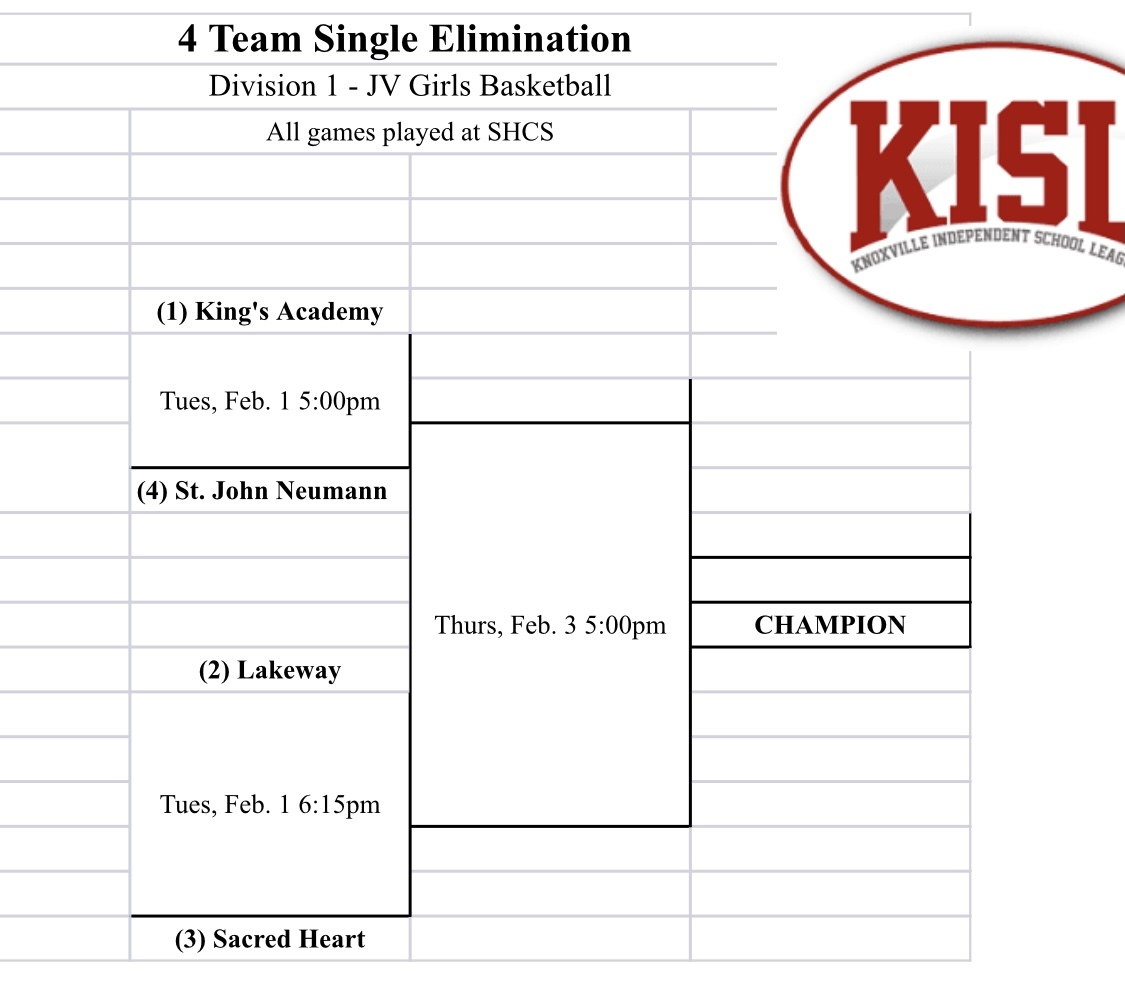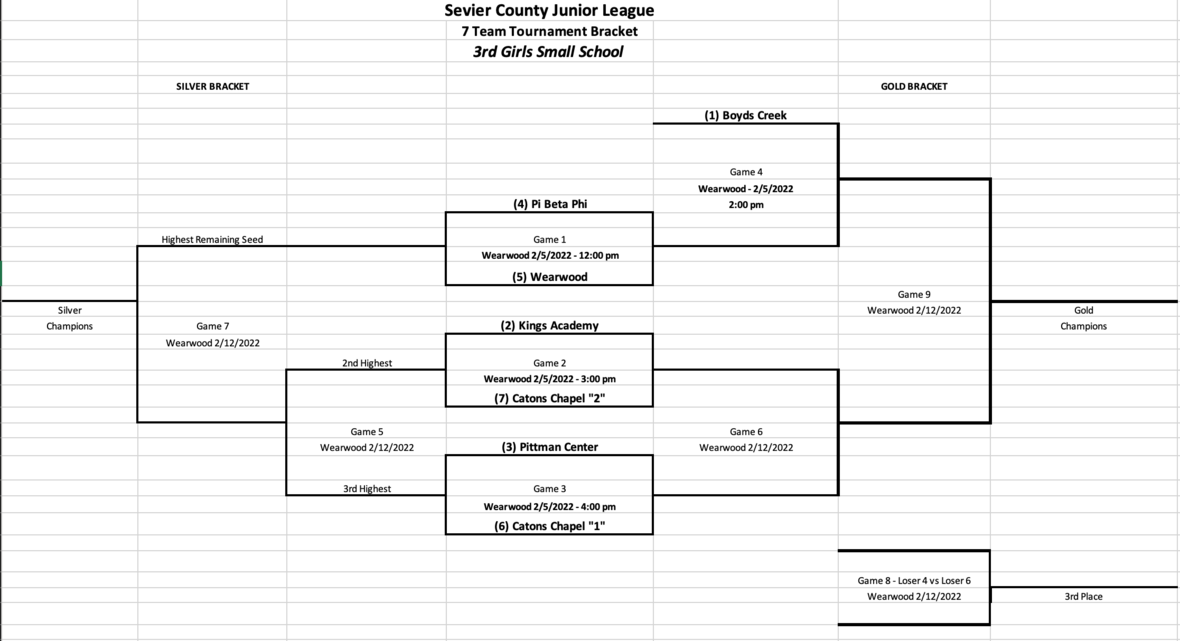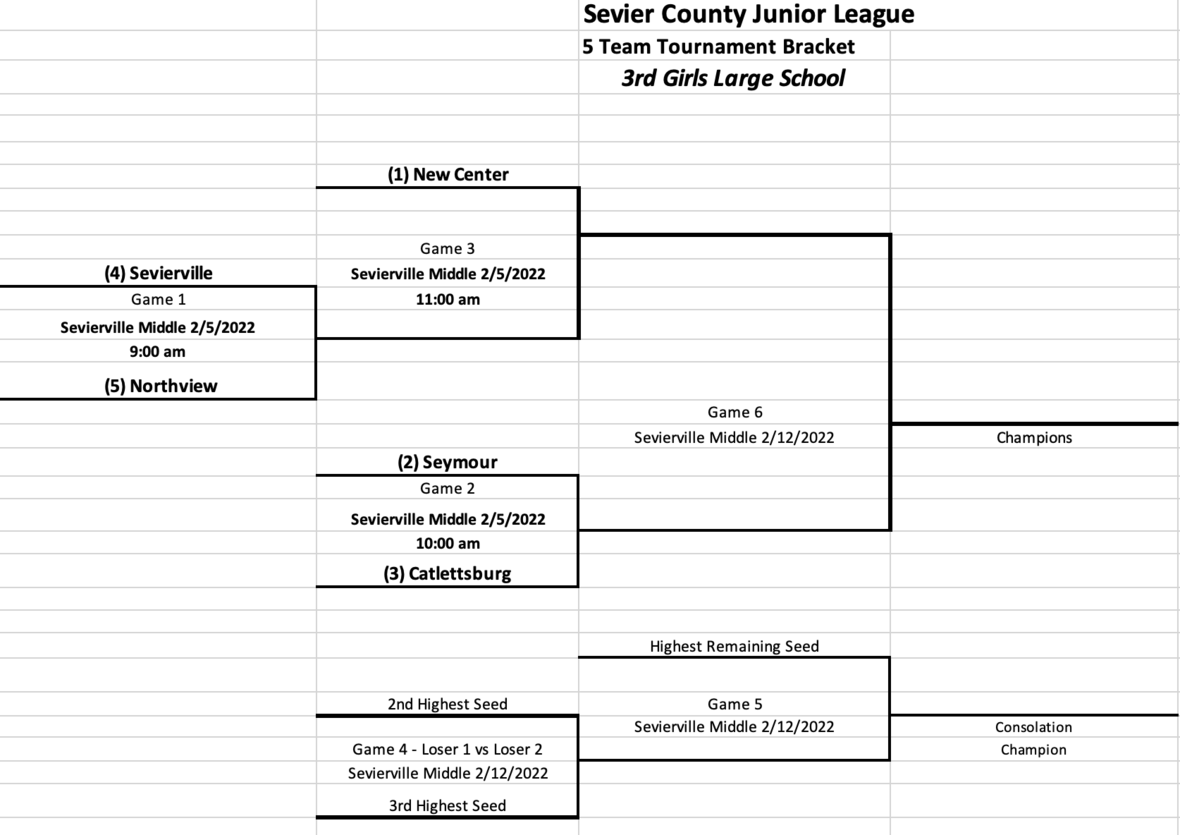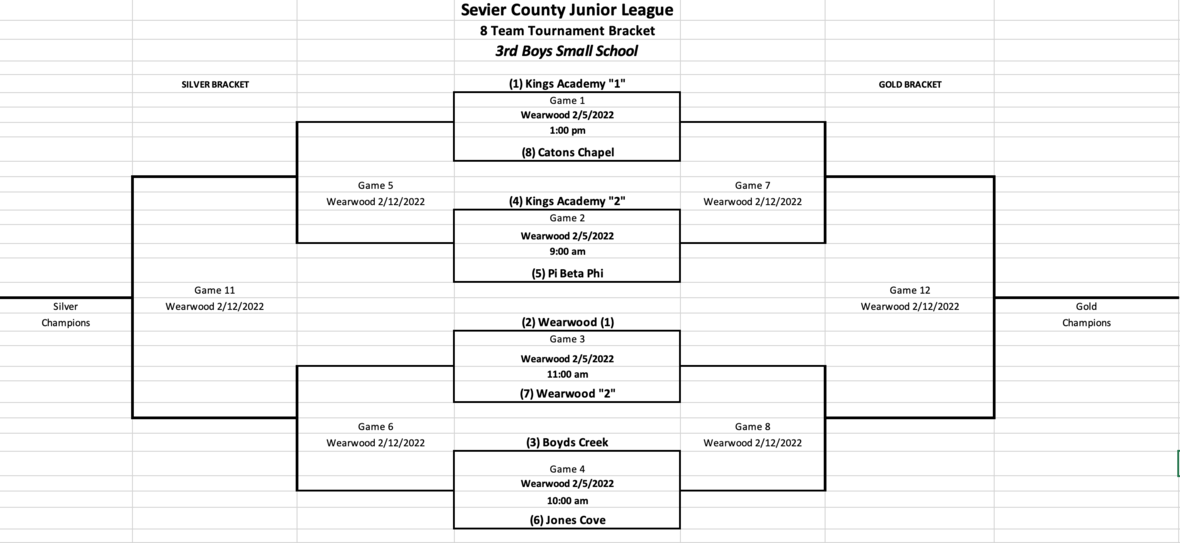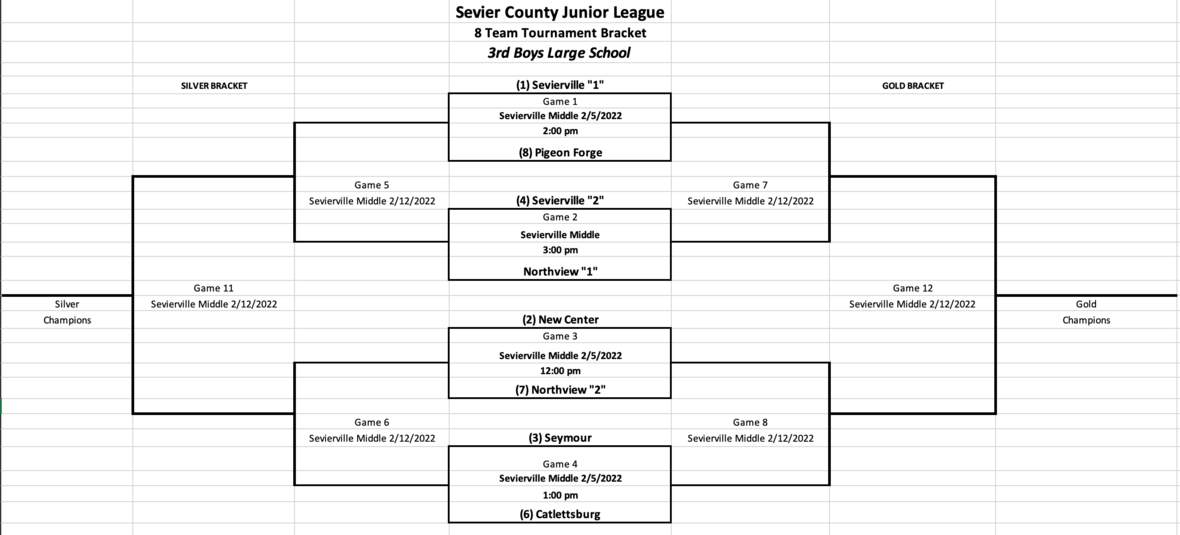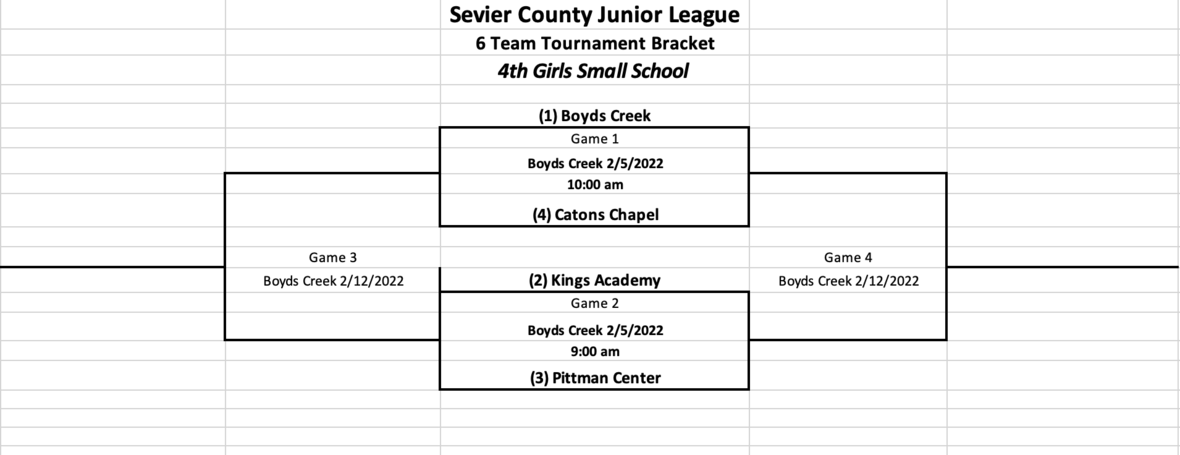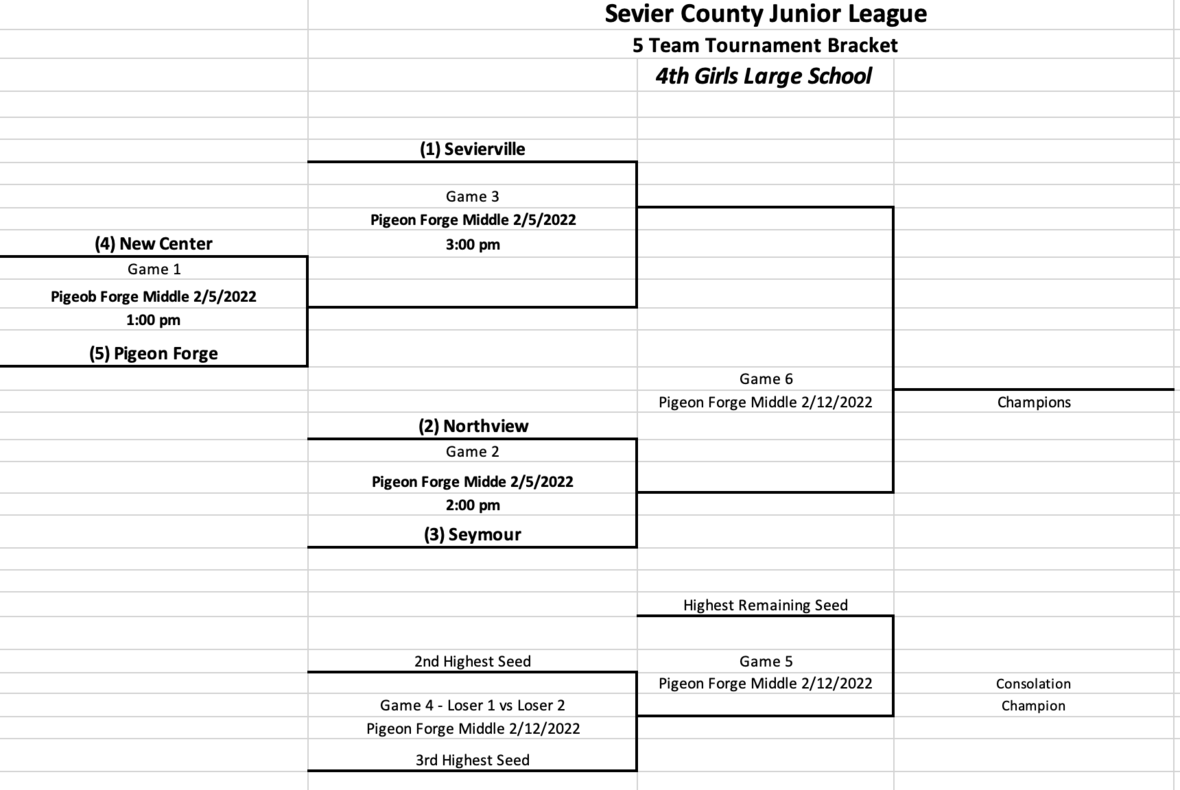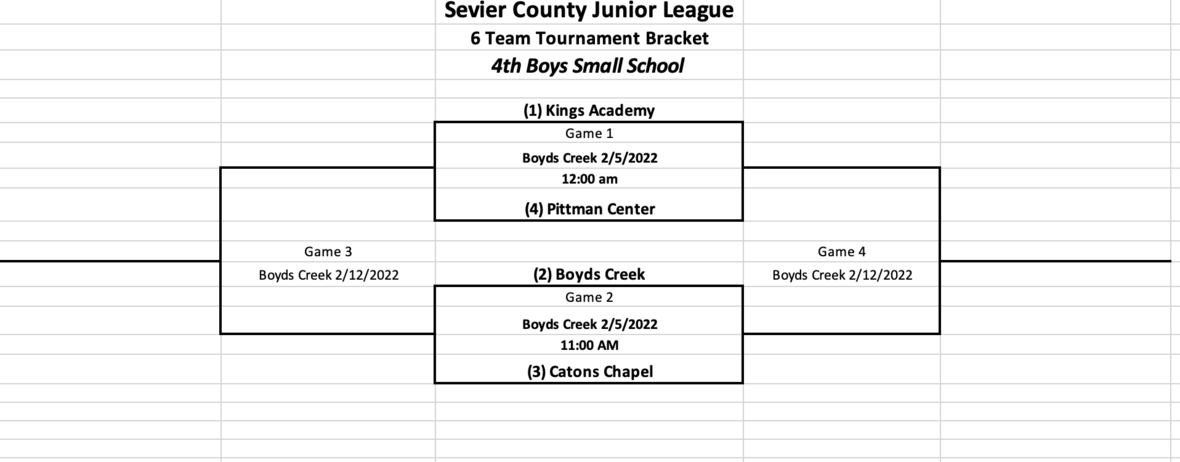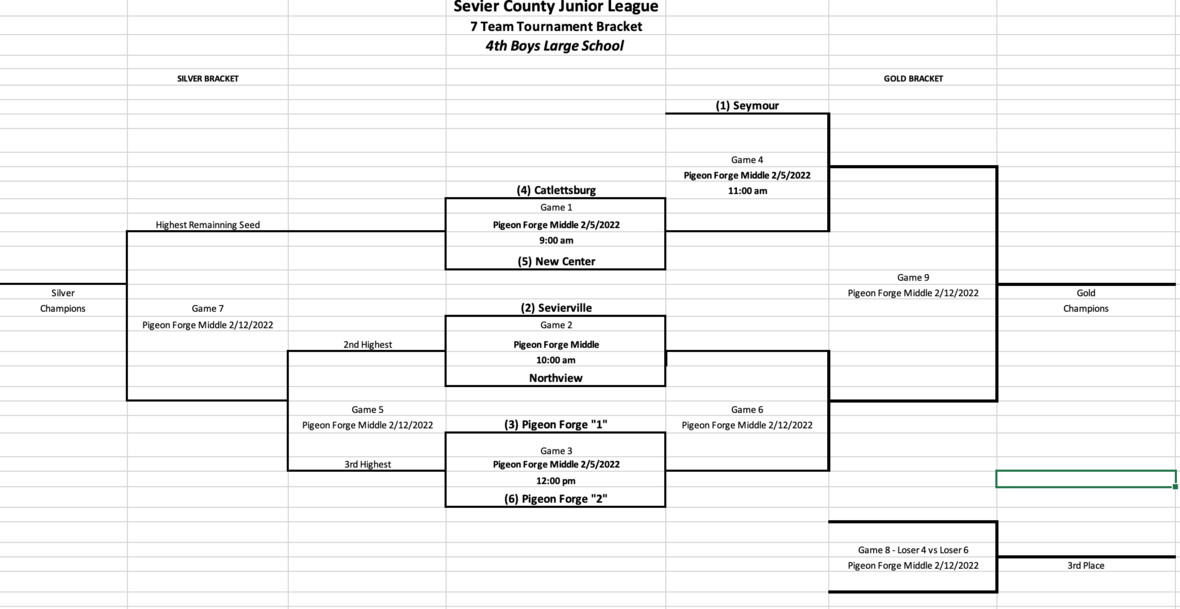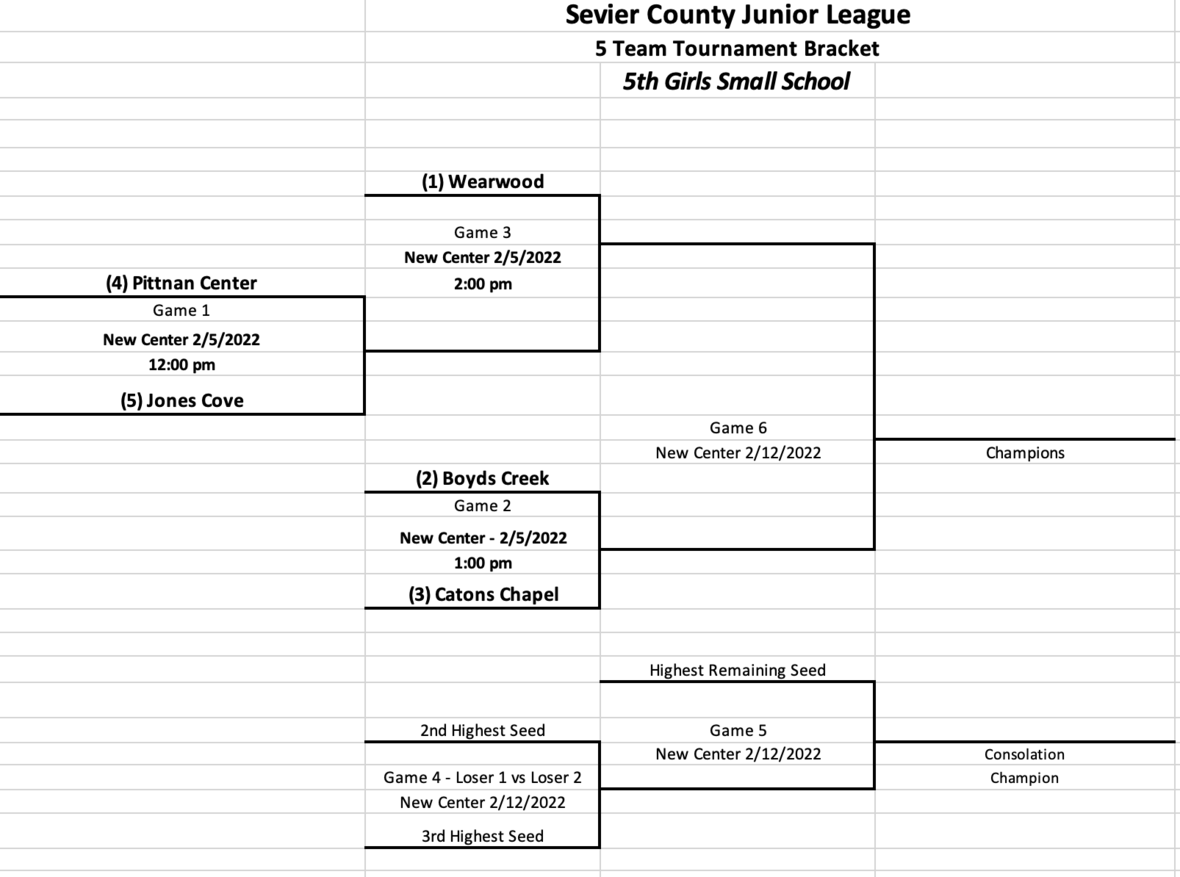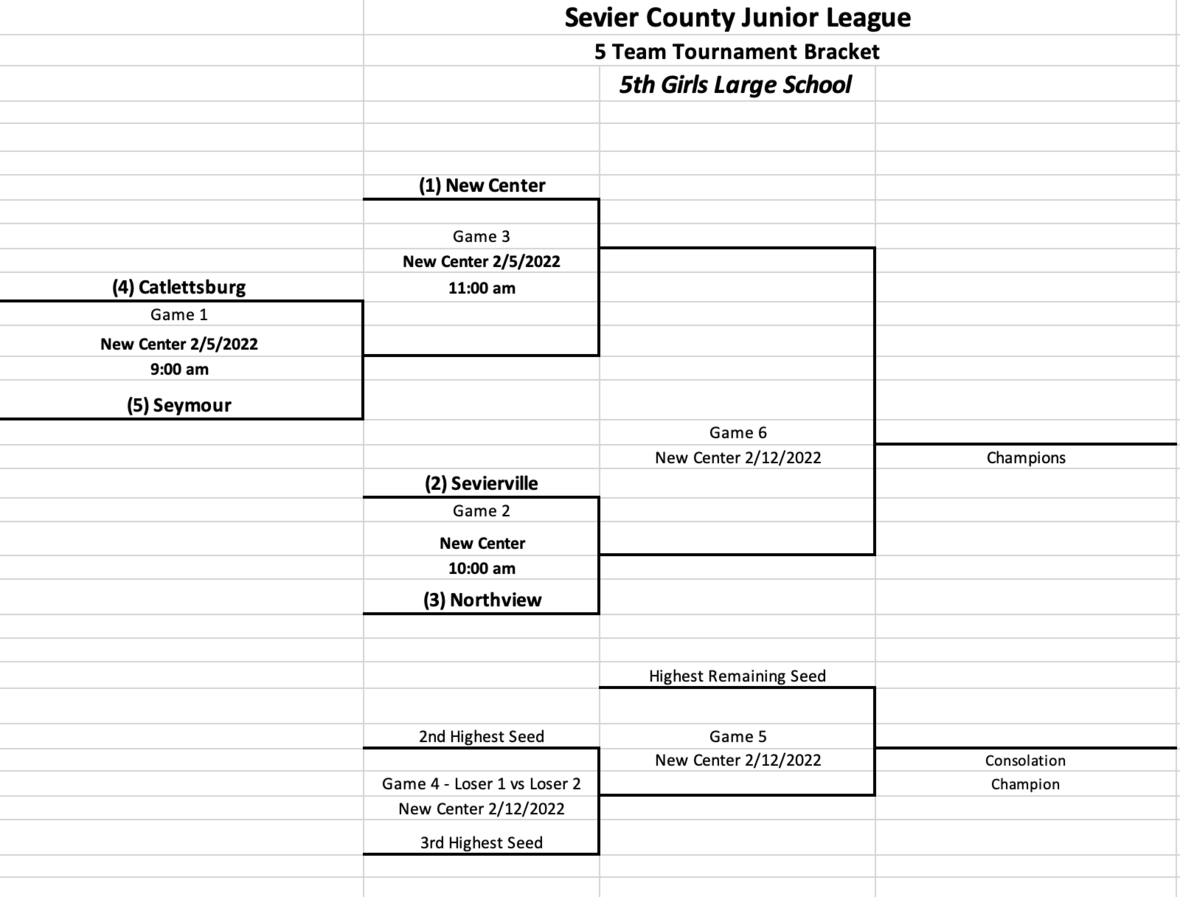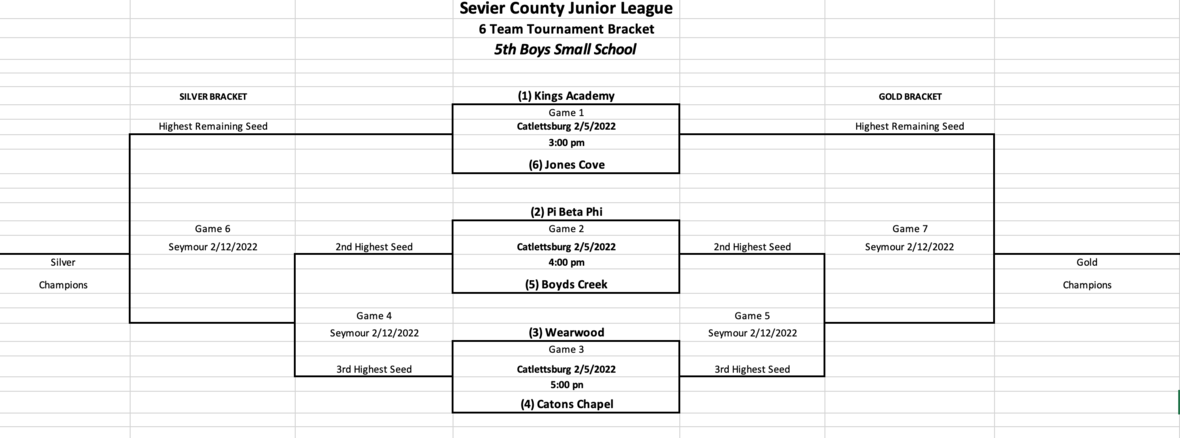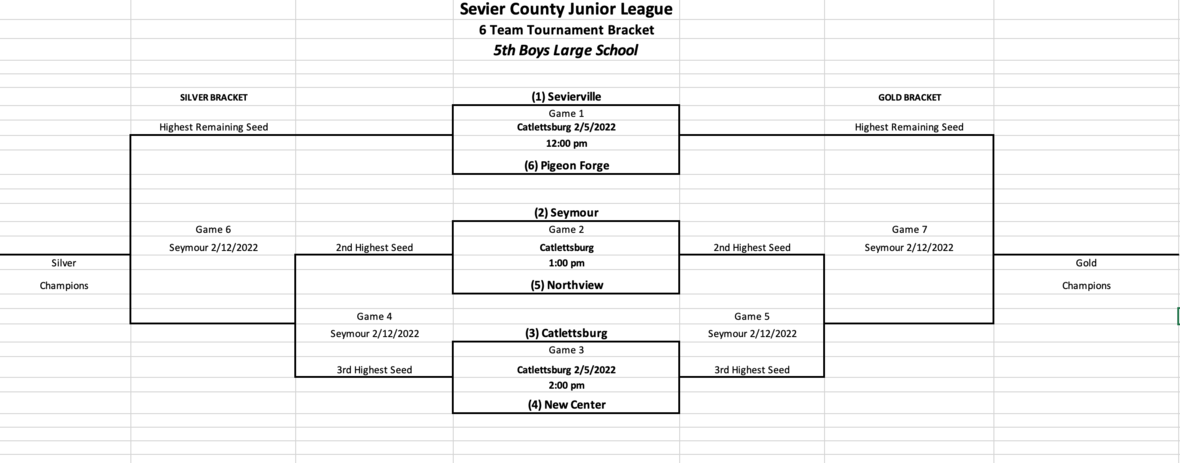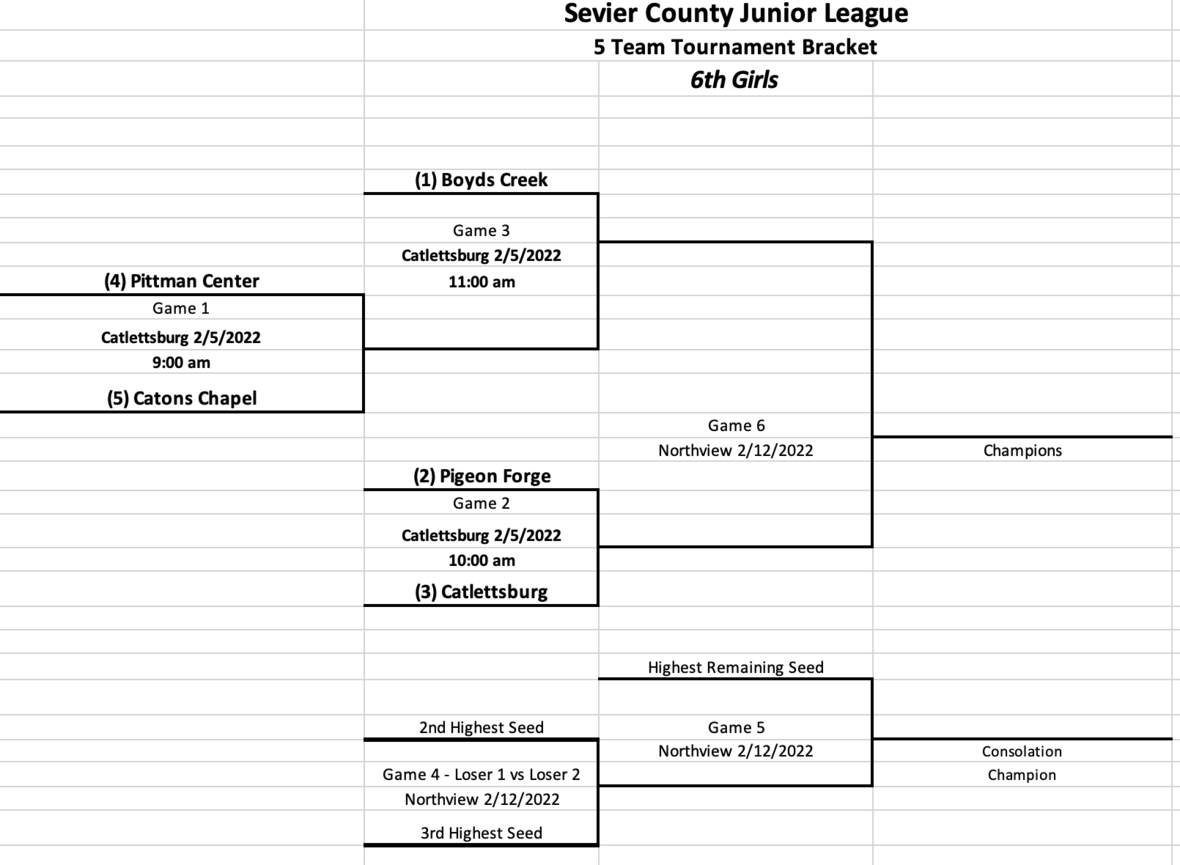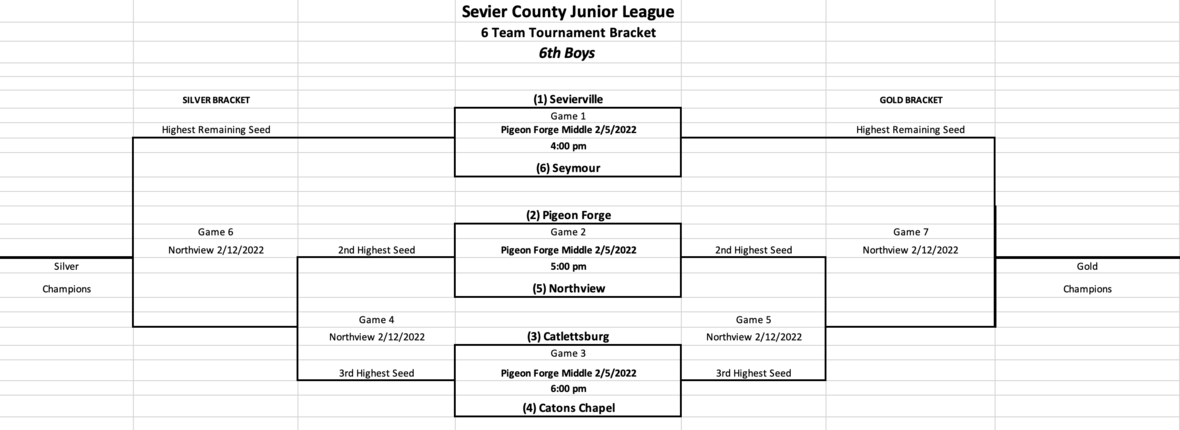table div table+table+table+table+table+table+table+table+table+table+table+table+table+table+table+table+table+table+table+table+table+table+table+table+table+table+table+table+table+table+table+table+table+table+table+table+table+table+table+table+table div table{width:100%;padding:0}table div table+table+table+table+table+table+table+table+table+table+table+table+table+table+table+table+table+table+table+table+table+table+table+table+table+table+table+table+table+table+table+table+table+table+table+table+table+table+table+table+table div table img{width:96.23%;padding:0;float:none}table div table+table+table+table+table+table+table+table+table+table+table+table+table+table+table+table+table+table+table+table+table+table+table+table+table+table+table+table+table+table+table+table+table+table+table+table+table+table+table+table+table div table td{width:100%;padding:0 1.88% 18px}/* styles */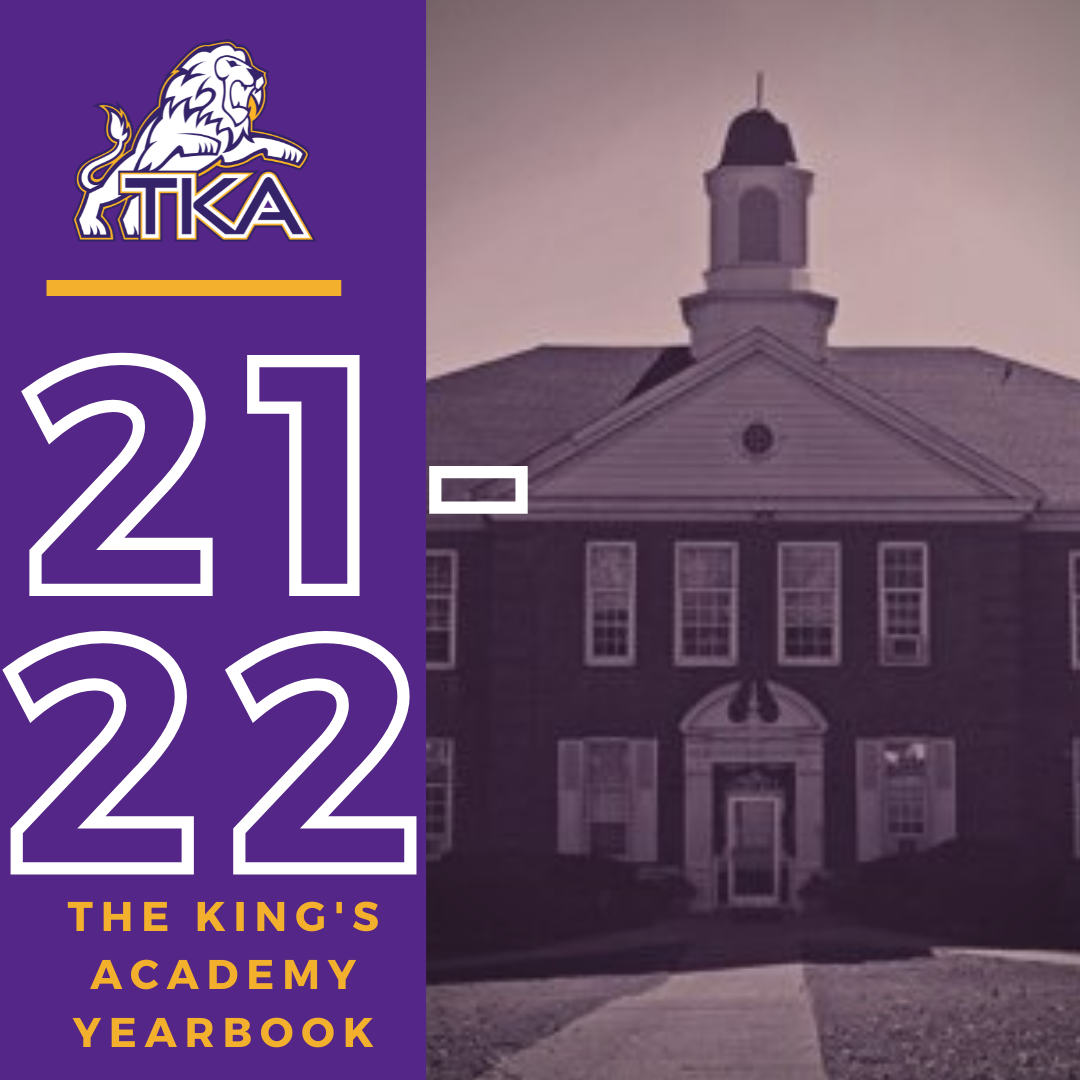# 2021-2022 Yearbook

The price for the 2021-2022 TKA yearbook is \$55. All grades, K3-12 will have the same yearbook. The slight price increase is because we are only going to have one larger all-school book.

All yearbook orders can be placed online--here is the link.Senior Tributes are available for purchase at the bottom of the link listed here.For any questions regarding yearbooks, email Mr. Josh Smith at jsmith@thekingsacademy.net.

 table div table+table+table+table+table+table+table+table+table+table+table+table+table+table+table+table+table+table+table+table+table+table+table+table+table+table+table+table+table+table+table+table+table+table+table+table+table+table+table+table+table+table+table+table+table div table{width:100%;padding:0}table div table+table+table+table+table+table+table+table+table+table+table+table+table+table+table+table+table+table+table+table+table+table+table+table+table+table+table+table+table+table+table+table+table+table+table+table+table+table+table+table+table+table+table+table+table div table img{width:96.23%;padding:0;float:none}table div table+table+table+table+table+table+table+table+table+table+table+table+table+table+table+table+table+table+table+table+table+table+table+table+table+table+table+table+table+table+table+table+table+table+table+table+table+table+table+table+table+table+table+table+table div table td{width:100%;padding:0 1.88% 18px}/* styles *//* styles */
 table div table+table+table+table+table+table+table+table+table+table+table+table+table+table+table+table+table+table+table+table+table+table+table+table+table+table+table+table+table+table+table+table+table+table+table+table+table+table+table+table+table+table+table+table+table+table+table+table div table{width:100%;padding:0}table div table+table+table+table+table+table+table+table+table+table+table+table+table+table+table+table+table+table+table+table+table+table+table+table+table+table+table+table+table+table+table+table+table+table+table+table+table+table+table+table+table+table+table+table+table+table+table+table div table img{width:96.23%;padding:0;float:none}table div table+table+table+table+table+table+table+table+table+table+table+table+table+table+table+table+table+table+table+table+table+table+table+table+table+table+table+table+table+table+table+table+table+table+table+table+table+table+table+table+table+table+table+table+table+table+table+table div table td{width:100%;padding:0 1.88% 18px}/* styles */# Covid-19 Procedures

While we are excited to offer in-person education, we must maintain a safe learning environment for all students, faculty/staff, and additional stakeholders.

To do so, we will be working closely with the Sevier County Health Department regarding Covid-19 protocols.

 table div table+table+table+table+table+table+table+table+table+table+table+table+table+table+table+table+table+table+table+table+table+table+table+table+table+table+table+table+table+table+table+table+table+table+table+table+table+table+table+table+table+table+table+table+table+table+table+table+table+table div table{width:100%;padding:0}table div table+table+table+table+table+table+table+table+table+table+table+table+table+table+table+table+table+table+table+table+table+table+table+table+table+table+table+table+table+table+table+table+table+table+table+table+table+table+table+table+table+table+table+table+table+table+table+table+table+table div table img{width:96.23%;padding:0;float:none}table div table+table+table+table+table+table+table+table+table+table+table+table+table+table+table+table+table+table+table+table+table+table+table+table+table+table+table+table+table+table+table+table+table+table+table+table+table+table+table+table+table+table+table+table+table+table+table+table+table+table div table td{width:100%;padding:0 1.88% 18px}/* styles */table div table+table+table+table+table+table+table+table+table+table+table+table+table+table+table+table+table+table+table+table+table+table+table+table+table+table+table+table+table+table+table+table+table+table+table+table+table+table+table+table+table+table+table+table+table+table+table+table+table+table+table+table div table{width:100%;padding:0}table div table+table+table+table+table+table+table+table+table+table+table+table+table+table+table+table+table+table+table+table+table+table+table+table+table+table+table+table+table+table+table+table+table+table+table+table+table+table+table+table+table+table+table+table+table+table+table+table+table+table+table+table div table img{width:96.23%;padding:0;float:none}table div table+table+table+table+table+table+table+table+table+table+table+table+table+table+table+table+table+table+table+table+table+table+table+table+table+table+table+table+table+table+table+table+table+table+table+table+table+table+table+table+table+table+table+table+table+table+table+table+table+table+table+table div table td{width:100%;padding:0 1.88% 18px}/* styles */